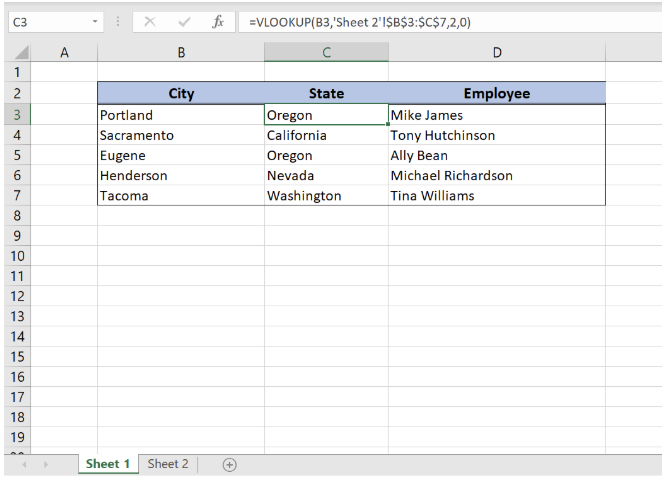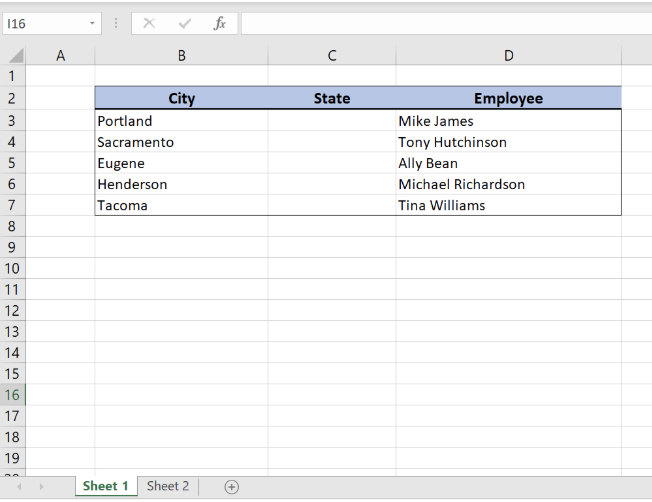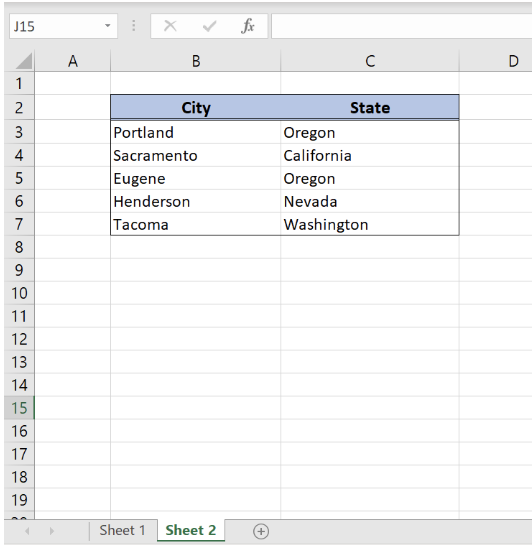Get instant live expert help with Excel or Google Sheets“My Excelchat expert helped me in less than 20 minutes, saving me what would have been 5 hours of work!”

#### Post your problem and you’ll get expert help in seconds.

Your message must be at least 40 characters
Our professional experts are available now. Your privacy is guaranteed.

# How to Pull Values from Another Worksheet in Excel

While working in Excel, we will often need to get values from another worksheet. This is possible by using the VLOOKUP function. In this tutorial, we will learn how to pull values from another worksheet in Excel, using VLOOKUP.Figure 1. Final result

## Syntax of the VLOOKUP formula

The generic formula for pulling values from another worksheet looks like:

`=VLOOKUP(lookup_value, ’sheet_name’!range, col_index_num, range_lookup)`

The parameters of the VLOOKUP function are:

• lookup_value – a value that we want to find in another worksheet
• ’sheet_name’!range – a range in another worksheet in which we want to lookup
• col_index_num – a column number in another worksheet from which we would like to pull a value
• range_lookup – default value 0. This means that we want to find an exact match for a lookup value.

## Setting up the DataFigure 2. “Sheet 1” in which we want to pull dataFigure 3. Sheet 2 from which we want to pull data

We will now look at the example to explain in detail how this function works. Above all, let’s start with examining the structure of the data that we will use.

In “Sheet 1” we have a table in which we want to pull data, while in “Sheet2” we have the table from which we want to pull data. “Sheet 1” consists of  “City” (column B), “State” (column C) and “Employee” (column D). “Sheet 2” consists of “City” (column B) and “State” (column C).

## Get the State from Sheet 2 using VLOOKUP

Our goal is to obtain data from the “State” column in the second worksheet and populate it into the “State” column of the 1st worksheet. This will be done based on each corresponding city.Figure 4. “Sheet 1” with pulled data in “State” column from “Sheet 2”

The formula looks like:

`=VLOOKUP(B3,'Sheet 2'!\$B\$3:\$C\$7,2,0)`

In our example, the lookup_value is the individual cell in “City” column. The parameter ’sheet_name’!range is ‘Sheet 2’!\$B\$3:\$C\$7 because we want to find value from the range B3:B7 in “Sheet 2”. Col_index_num has value 2, as we want to pull value from the second column of the range. Finally, range_lookup has value 0, because we want to find an exact match of “City” values.

Please note that we put absolute cell reference in table range (\$ before B3:C7 range) as we must fix our lookup table.

To pull values from another worksheet, we need to follow these steps:

• Select cell C3 and click on it
• Insert the formula: `=VLOOKUP(B3,'Sheet 2'!\$B\$3:\$C\$7,2,0)`
• Press enter
• Drag the formula down to the other cells in the column by clicking and dragging the little “+” icon at the bottom-right of the cell.

As a result, we will get Oregon state in the cell B3. As you can see, the value of “City” in B3 on the “Sheet 1” is Portland, while in the “Sheet 2” state for Portland is Oregon. The function pulls this value and returns it to “Sheet 1” as a result.

## Instant Connection to an Expert through our Excelchat Service:

Most of the time, the problem you will need to solve will be more complex than a simple application of a formula or function. If you want to save hours of research and frustration, try our live Excelchat service! Our Excel Experts are available 24/7 to answer any Excel question you may have. We guarantee a connection within 30 seconds and a customized solution within 20 minutes.

Are you still looking for help with the VLOOKUP function? View our comprehensive round-up of VLOOKUP function tutorials here.

### Did this post not answer your question? Get a solution from connecting with the expert.Another blog reader asked this question today on Excelchat:
Solution examplesI have a pivot table in which per order (on the rows) stands how much products they ordered per size (on the columns). I want to determine which combinations of quantities of sizes people order. And I want to count these combinations.
Solved by E. W. in 60 minsI have 500 numbers in column A with aproximatly 15digits. In column B I have 5 numbers with 6 digits Can a find a formula wich can transfer all numbers from A column wich start with some 6 digitsa from B column
Solved by V. L. in 32 minsI have a sheet with 3 columns. First column is a code for records in column B (has 563 records). What I need is a formula to show me if what is in column C (has 4400 records) is in column B and if it is to take the code for that record. Example: Column A(code corresponding to column B): 12, 14, 15, 19 Column B(names): asd, adf, ade, aqw Column C(names): akd, adf, ade, anb, wgs I need something that would say, if record in column C (for example adf) is matching record in column B (adf) return the code from column A (the code corresponding to that record in column B),if not return blank.
Solved by C. J. in 8 minsIf the data in A matches the data in C, then I need the data in E to be placed in B
Solved by X. W. in 40 minsI have a workbook with 2 sheets. First sheet has column A which is a device name, then columns B - infinity that have numeric "tags" for that device. Each device can have one or 20 "tags", tags are all numerical. Worksheet 2 has column A which is numeric value and B which is a human readable value of what the tag is. Question is, how do I replace all the tag numbers in worksheet 1 with the actual human readable values is sheet 2?
Solved by T. H. in 16 mins

### Leave a CommentGuest
rahul sharma

Sir

I need to pull data form sheet to another sheet
in first sheet 58 items with there price, qty, make, installation charges, consideration(yes/No) etc.
in second sheet i need to pull only those rows which I have considered yes.

example:

if i have considered (yes ) for item no. 2, 5, 6, 8, 13, 15, 17, 22, 28, etc. then only these items (Row with their price, qty, Installation charge, make, model etc.) will be reflect in second sheet.

Comment awaiting moderation

## Subscribe to Excelchat.co

Get updates on helpful Excel topics

## Did this post not answer your question? Get a solution from connecting with the expertAnother blog reader asked this question today on Excelchat:

#### Post your problem and you’ll get expert help in seconds.

Your message must be at least 40 characters
Our professional experts are available now. Your privacy is guaranteed.
Trusted by people who work at